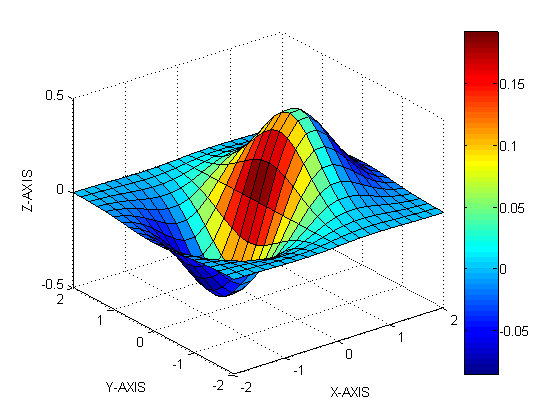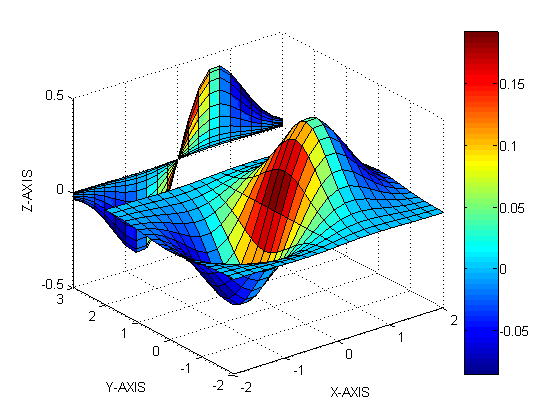3-D VisualizationExample -- Displaying Cross-Sections of Surfaces

Sometimes projecting a 3D surface onto an x-, y-, or z-axis can aid visualization. To do this, you might change the aspect ratio, in order to make space for the projection. The following example illustrates how to do this:

1. Create an x-y grid and z-values for it:
• ```[x,y] = meshgrid([-2:.2:2]);
Z = x.*exp(-x.^2-y.^2);
```
2. Plot the surface in 3-D; annotate with a colorbar and axis labels:
• ```surf(x,y,Z,gradient(Z))
colorbar
xlabel('X-AXIS')
ylabel('Y-AXIS')
zlabel('Z-AXIS')```
3. Use `axis` to change the `Ymax` value in to 3, stretching the plot in one direction:
• ```axis([-2 2 -2 3 -0.5 0.5]) %
```
4. Regrid the surface, setting all Y-values equal to `3`:
• ```y = 3*ones(21);
```
5. Plaster a plot of the surface onto the Y-axis:
• ```hold on
surf(x,y,Z,gradient(Z))```Effects of Setting Aspect Ratio Properties Example -- Displaying Real Objects© 1994-2005 The MathWorks, Inc.